# Post Correspondence Problem

Post Correspondence Problem is a popular undecidable problem that was introduced by Emil Leon Post in 1946. It is simpler than Halting Problem.

In this problem we have N number of Dominos (tiles). The aim is to arrange tiles in such order that string made by Numerators is same as string made by Denominators.
In simple words, lets assume we have two lists both containing N words, aim is to find out concatenation of these words in some sequence such that both lists yield same result.

Let’s try understanding this by taking two lists A and B

`A=[aa, bb, abb] and B=[aab, ba, b] `

Now for sequence 1, 2, 1, 3 first list will yield aabbaaabb and second list will yield same string aabbaaabb.
So the solution to this PCP becomes 1, 2, 1, 3.

Post Correspondence Problems can be represented in two ways:

1. Domino’s Form :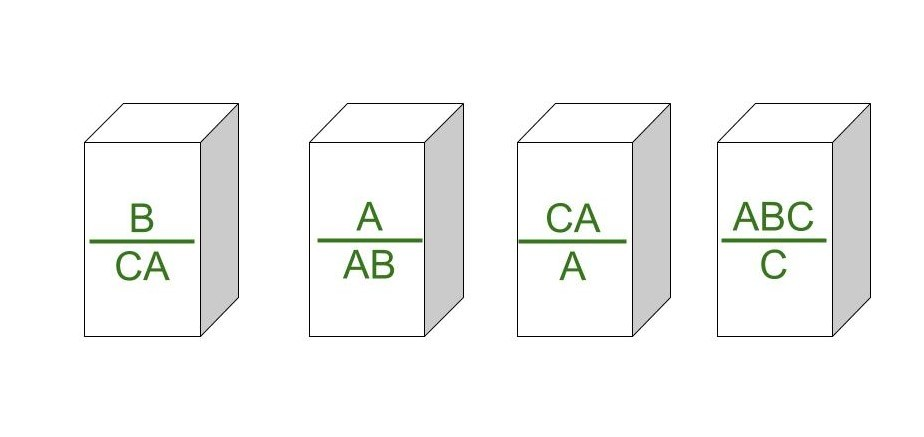2. Table Form :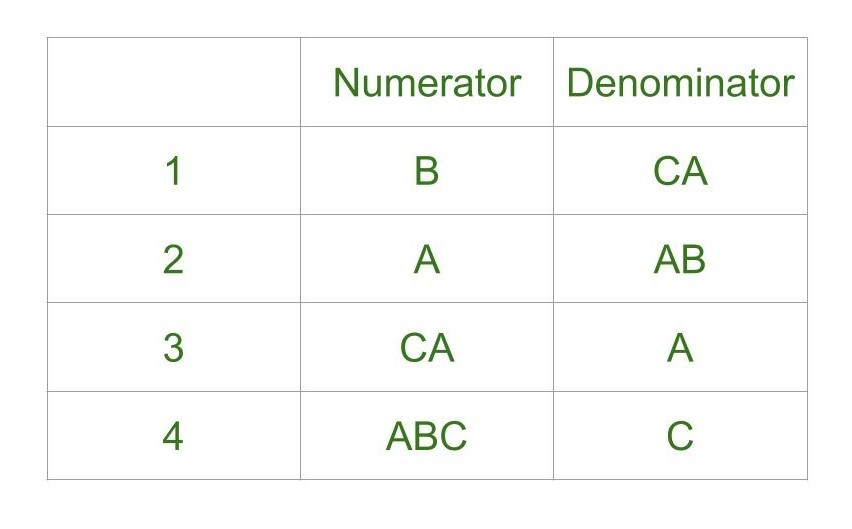Lets consider following examples.

Example-1: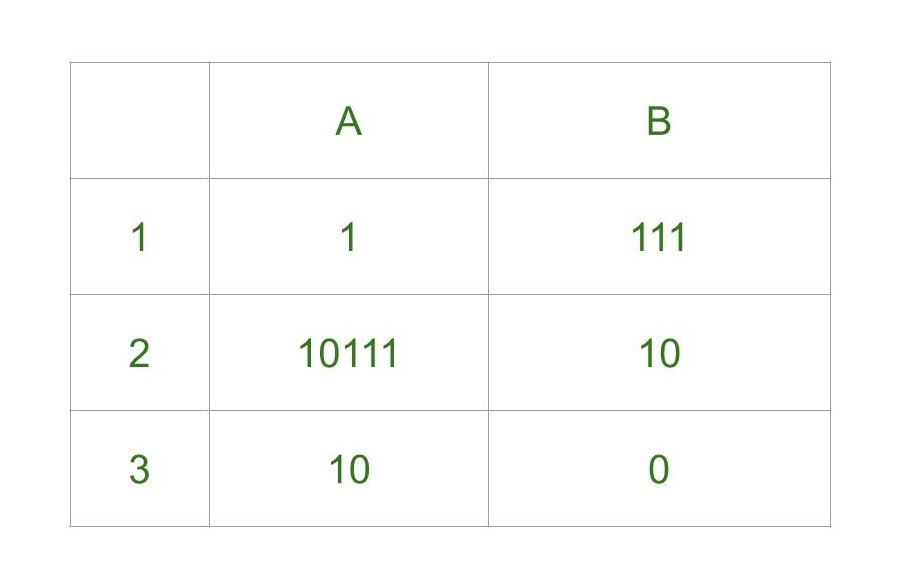Explanation –

• Step-1:
We will start with tile in which numerator and denominator are starting with same number, so we can start with either 1 or 2.
Lets go with second tile, string made by numerator- 10111, string made by denominator is 10.

• Step-2:
We need 1s in denominator to match 1s in numerator so we will go with first tile, string made by numerator is 10111 1, string made by denominator is 10 111.

• Step-3:
There is extra 1 in numerator to match this 1 we will add first tile in sequence, string made by numerator is now 10111 1 1, string made by denominator is 10 111 111.

• Step-4:
Now there is extra 1 in denominator to match it we will add third tile, string made by numerator is 10111 1 1 10, string made by denominator is 10 111 111 0.

```Final Solution - 2 1 1 3
String made by denominators: 101111110 ```

As you can see, strings are same.

Example-2: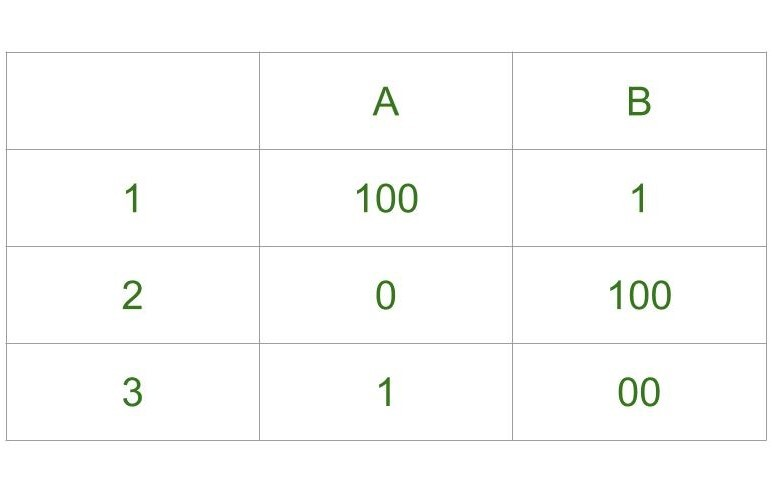Explanation –

• Step-1:
We will start from tile 1 as it is our only option, string made by numerator is 100, string made by denominator is 1.

• Step-2:
We have extra 00 in numerator, to balance this only way is to add tile 3 to sequence, string made by numerator is 100 1, string made by denominator is 1 00.

• Step-3:
There is extra 1 in numerator to balance we can either add tile 1 or tile 2. Lets try adding tile 1 first, string made by numerator is 100 1 100, string made by denominator is 1 00 1.

• Step-4:
There is extra 100 in numerator, to balance this we can add 1st tile again, string made by numerator is 100 1 100 100, string made by denominator is 1 00 1 1 1. The 6th digit in numerator string is 0 which is different from 6th digit in string made by denominator which is 1.

We can try unlimited combinations like one above but none of combination will lead us to solution, thus this problem does not have solution.

Undecidability of Post Correspondence Problem :
As theorem says that PCP is undecidable. That is, there is no particular algorithm that determines whether any Post Correspondence System has solution or not.

Proof –
We already know about undecidablitiy of Turing Machine. If we are able to reduce Turing Machine to PCP then we will prove that PCP is undecidable as well.

Consider Turing machine M to simulate PCP’s input string w can be represented as .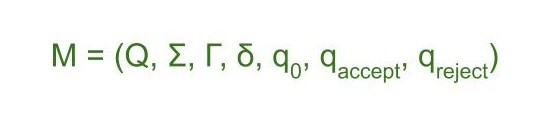If there is match in input string w, then Turing machine M halts in accepting state. This halting state of Turing machine is acceptance problem ATM.
We know that acceptance problem ATM is undecidable. Therefore PCP problem is also undecidable.

To force simulation of M, we make 2 modifications to Turing Machine M and one change to our PCP problem.

1. M on input w can never attempt to move tape head beyond left end of input tape.
2. If input is empty string € we use _ .
3. PCP problem starts match with first domino [u1/v1] This is called Modified PCP problem.
`MPCP = {[D] | D is instance of PCP starts with first domino} `

Construction Steps –

1. Put [# / (#q0w1w2..wn#)] into D as first domino, where is instance of D is MPCP. A partial match is obtained in first domino is # at one face is same #symbol in other face.
2. Transition functions for Turing Machine M can have moves Left L, Right R. For every x, y z in tape alphabets and q, r in Q where q is not equal to qreject. If transition(q, x) = (r, y, R) put domino [qx / by] into D and transition(q, x) =(r, y, L) put domino [zqx / rzy] into D.
3. For every tape alphabet x put [x / x] into D.To mark separation of each configurations put [# / #] and [# / _#] into D .
4. To read input alphabets x even after Turing Machine is accepting state put [xqa / qa] and [qax / qa]and [qa# / #] into D. These steps concludes construction of D.

Since this instance of MPCP, we need to convert this to PCP. So to convert to D, we consider below domino and strings matching.

Converting MPCP To PCP :
Let u = u1, u2, …, un be any string of input length n and modify these strings as

```\$u = *u1*u2*u3* …*un
u\$ = u1* u2* u3* … un*
\$u\$ = * u1* u2* u3* ... un* ```

Let D be set of two faced Dominos,

`D = {[u1 / v1], [u2 / v2], [u3 / v3], ..., [un / vn]} and {[\$u1 / \$v1\$], [\$u2 / v2\$], ..., [*_ / _]} `

From above dominos collection, we could see only domino has partial match starts with [\$u1 / \$v1\$] and to place marker end of inputs[*_ / _].
There by we can avoid stating explicit requirement of domino should start with first domino.
If number of configurations of Turing machine does not lie within value of qngn, then Turing machine is looping state. It does not halt.

Attention reader! Don’t stop learning now. Get hold of all the important CS Theory concepts for SDE interviews with the CS Theory Course at a student-friendly price and become industry ready.

My Personal Notes arrow_drop_up

## Recommended Posts:Check out this Author's contributed articles.

If you like GeeksforGeeks and would like to contribute, you can also write an article using contribute.geeksforgeeks.org or mail your article to contribute@geeksforgeeks.org. See your article appearing on the GeeksforGeeks main page and help other Geeks.

Please Improve this article if you find anything incorrect by clicking on the "Improve Article" button below.

Article Tags :

Be the First to upvote.

Please write to us at contribute@geeksforgeeks.org to report any issue with the above content.